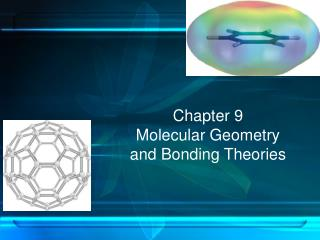# Chapter 9 Molecular Geometry and Bonding Theories - PowerPoint PPT PresentationDownload PresentationChapter 9 Molecular Geometry and Bonding Theories

Chapter 9 Molecular Geometry and Bonding TheoriesDownload Presentation## Chapter 9 Molecular Geometry and Bonding Theories

- - - - - - - - - - - - - - - - - - - - - - - - - - - E N D - - - - - - - - - - - - - - - - - - - - - - - - - - -
##### Presentation Transcript

1. Chapter 9Molecular Geometryand Bonding Theories

2. Molecular Shapes • Shape of a molecule plays an important role in its reactivity • The number of bonding and nonbonding electron pairs is used to predict the shape of the molecule Lewis structure of carbon tetrachloride, CCl4

3. Fig 9.1 Tetrahedral geometry of CCl4

4. Fig 9.2 Some common molecular shapes

5. Fig 9.3 Shapes of ABn molecules

6. Fig 9.3 Derivatives from ABn geometry CH4 NH3 H2O

7. What Determines the Shape of a Molecule? • Electron pairs, whether they be bonding or nonbonding, repel each other • By assuming the electron pairs are placed as far as possible from each other, we can predict the shape of the molecule

8. Electron Domains • Electron pairs are referred to as electron domains. • In a double or triple bond, all electrons shared between those two atoms are on the same side of the central atom; therefore, they count as one electron domain. • Central atom, A, in this molecule, has four electron domains

9. Valence shell electron pair repulsion (VSEPR) model: The best arrangement of a given number of electron domains is the one that minimizes the repulsions among them. Fig 9.5 Balloon analogy for electron domains

10. Table 9.1 Electron-domain geometries as a function of the number of electron domains

11. Fig 9.6 The molecular geometry of NH3 • Electron-domain geometry is often not the shape of the molecule, however. • Molecular geometry is that defined by the positions of only the atoms in the molecules, not the nonbonding pairs.

12. The molecular geometry of CO2 Linear; 180° The molecular geometry of O3 Bent; 120°

13. 0 lone pairs on central atom Cl Be Cl 2 atoms bonded to central atom BeCl2

14. BF3

15. CH4

16. PCl5 axial equatorial axial

17. SF6

18. Linear Electron Domain Table 9.2 • In the linear domain, there is only one molecular geometry: linear • NOTE: If there are only two atoms in the molecule, the molecule will be linear no matter what the electron domain

19. Trigonal Planar Electron Domain Table 9.2 • There are two molecular geometries: • Trigonal planar, if all the electron domains are bonding • Bent, if one of the domains is a nonbonding pair

20. Tetrahedral Electron Domain Table 9.2 • There are three molecular geometries: • Tetrahedral, if all are bonding pairs, • Trigonal pyramidal if one is a nonbonding pair, • Bent if there are two nonbonding pairs.

21. Nonbonding Pairs and Bond Angles • Nonbonding pairs are physically larger than bonding pairs. • Therefore, their repulsions are greater; this tends to decrease bond angles in a molecule.

22. Multiple Bonds and Bond Angles • Double and triple bonds place greater electron density on one side of the central atom than do single bonds: • Therefore, they also affect bond angles.

23. Trigonal Bipyramidal Electron Domain • There are two distinct positions in this geometry: • Axial • Equatorial

24. Trigonal Bipyramidal Electron Domain SF4 Lower-energy conformations result from having nonbonding electron pairs in equatorial, rather than axial, positions in this geometry.

25. Trigonal Bipyramidal Electron Domain Table 9.3 • There are four distinct molecular geometries in this domain: • Trigonal bipyramidal • Seesaw • T-shaped • Linear

26. Octahedral Electron Domain Table 9.3 • All positions are equivalent in the octahedral domain. • There are three molecular geometries: • Octahedral • Square pyramidal • Square planar

27. What are the molecular geometries of SO2 and SF4? F S F F O O S F Predicting Molecular Geometry • Draw Lewis structure for molecule. • Count number of lone pairs on the central atom and number of atoms bonded to the central atom. • Use VSEPR to predict the geometry of the molecule. AB4E AB2E distorted tetrahedron bent